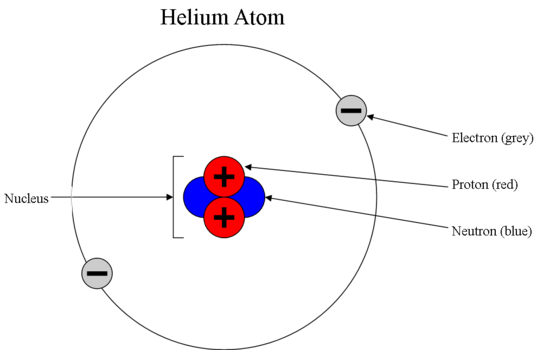# Introduction to Electron## What are Electrons?

Electrons are subatomic particles that hold an elementary charge of magnitude -1. The charge of an electron is equal in magnitude to the charge held by a proton (but has an opposite sign). Therefore, electrically neutral atoms/molecules must have an equal number of electrons and protons. Although the magnitude of the charges held by protons and electrons are the same, the size and mass of an electron are much smaller than that of a proton (the mass of an electron is roughly 1/1836 the mass of a proton).

## Charge of Electrons

An electron is a negatively charged particle. The negative charge is equal to 1.602 × 10-19 coulomb in magnitude. The mass of an electron is 1/1837 of a proton.

## Mass of Electrons

The mass of an electron is 9.10938356 × 10-31 kilograms. The mass of the electron is negligible compared to the mass of the proton.

Owing to their small size and mass, the properties exhibited by electrons can be studied better with the help of quantum mechanics rather than classical mechanics. This is because matter behaves differently at the quantum scale. For example, the uncertainty associated with the position and the velocity of an electron is much greater than that associated with a proton or a neutron, as per Heisenberg’s uncertainty principle. Electrons are distributed around the nuclei of atoms in atomic orbitals, which can be simply visualized as regions around the nucleus in which the probability of finding a specific electron is the highest.

### Who Discovered the Electron?

The electron was discovered by the English physicist J.J.Thomson in the year 1897, via his experiments with cathode ray tubes.Electrons – The Sub-atomic Particles